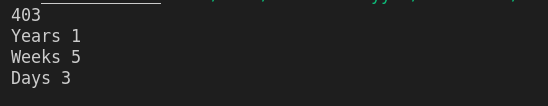Write a c++ program that takes as input days as an from the user shows the output in years, weeks and days

Sample Input:

403

Sample Output

Years 1

Weeks 5

Days 3

#include <iostream> //Preprogressive Directive
using namespace std; // Global Identifier

int main() // Main Function
{
int days, week = 7, year = 365;
cin >> days;
int years = days / year;
days = days % year;
int weeks = days / week;
days = days % week;

cout << "Years " << years << endl;
cout << "Weeks " << weeks << endl;
cout << "Days " << days << endl;

return 0;
}c++ programming exercise with solution